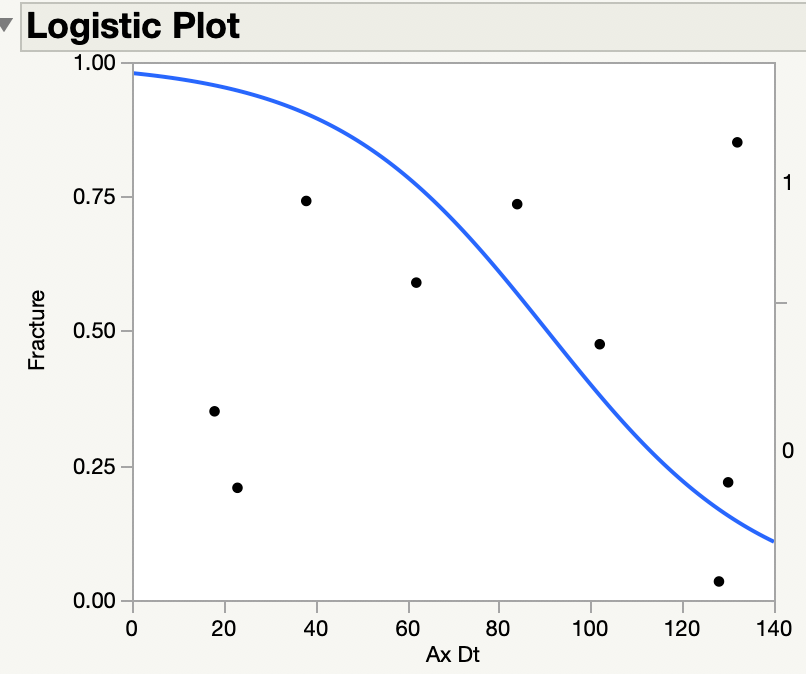Choose Language Hide Translation Bar
Highlighted

## Weibull Regression for risk curve

Hi evryone!

I have a set of data with 2 variables: one is binary (f: y/n) the other one continu (a).

The higher "a", the more chance to have f=yes.

I need to use the Weibull regression to find the 0.5 probability to get f=yes. This is like a logistic regression, but one of the advantages of using the weibull is that the risk curve goes through 0 and 1, unlike the logistic regression.

I found a Weibull tool in the fit curve menu, but the curve stops at .8...

Can someone help me?

thanks

4 REPLIES 4
Highlighted

## Re: Weibull Regression for risk curve

I don't know what you mean by "the (Weibull) risk curve goes through 0 and 1, unlike the logistic regression." Do you mean that you are using a Weibull CDF to model the response? Are you referring to the values (0,1) that you use to code the binary levels or the probability of fracture?

The regression will not necessarily achieve 0 or 1 if the range of the predictor is limited such that the asymptotes are not achieved. I tried to read the Ax Dt values from the plot and then performed a nominal logistic regression.You do not need to achieve the limiting 0 and 1 asymptotes over the observed range to apply inverse prediction to estimate Ax Dt for probability = 0.5.

The shape of the curve is constrained by the function you use for your model. The binary nature forces a transition from one asymptote to the other. The higher asymptote is limited to 0.8 because 4 out of 5 responses are 1 and 1 out of 5 is 0. This observation will also limit the logistic curve.

Learn it once, use it forever!
Highlighted

## Re: Weibull Regression for risk curve

"Do you mean that you are using a Weibull CDF to model the response? Are you referring to the values (0,1) that you use to code the binary levels or the probability of fracture?"

Yes absolutly!

I understand the 0.8 asymptot limit, but I have seen numeral models representations going from 0 to 1 even with data not totaly separated by a treshold.

Is there a way to "force" the weibull cdf fitting to go from 0 to 1?

in the mean time, ho can I calculate the .5 risk with the logistic regression?

BTW: Happy new year!

Highlighted

## Re: Weibull Regression for risk curve

Happy new year!

The Weibull CDF goes from 0 to 1. The fitted function does, too. The plot does not show these limits because that plot would entail extrapolation beyond the domain of the observations. Also, regardless of the plot, achieving 0 or 1 with the data is not essential to estimating the Ax Dt for Pr( Y=Fracture ) = 0.5.

Use the Analyze > Fit Model for the logistic regression. Then click the red triangle at the top and select Inverse Prediction. Enter 0.5 for the response value and select any other paramters as desired.

See Help > Books > Fitting Linear Models and the chapter on Nominal Logistic Regression for more details and examples.

Learn it once, use it forever!
Highlighted

## Re: Weibull Regression for risk curve

I'm ok for p=0.5

However, plotting the weibull CDF clearly shows it will not go to 1...
Article Labels

There are no labels assigned to this post.